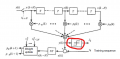# How to implement a Decision Device,in matlab ?

#### kalemaxon89

Joined Oct 12, 2022
91
I am implementing this linear equaliser in matlab which equalises a 4-PSK containing ISI.

However, I cannot figure out how to implement the "decision device" block (circled in red in the figure).
It decides its output based on whether the amplitude of the quantized pulse and the noise, exceeds a pre-determined value or not ..
but I do not understand how to write it in code.

I am thinking for example to define the ideal decision levels of the 4-PSK decision_levels = [1+1i, 1-1i, -1+1i, -1-1i]; and then compare them with y(k) with a couple of if-else.Ideas? Suggestions?
Is there already a matlab function that does this?

Thanks!

Last edited by a moderator: